# `sklearn.linear_model`.Ridge¶

class `sklearn.linear_model.``Ridge`(alpha=1.0, fit_intercept=True, normalize=False, copy_X=True, max_iter=None, tol=0.001, solver=’auto’, random_state=None)[source]

Linear least squares with l2 regularization.

This model solves a regression model where the loss function is the linear least squares function and regularization is given by the l2-norm. Also known as Ridge Regression or Tikhonov regularization. This estimator has built-in support for multi-variate regression (i.e., when y is a 2d-array of shape [n_samples, n_targets]).

Read more in the User Guide.

Parameters: alpha : {float, array-like}, shape (n_targets) Regularization strength; must be a positive float. Regularization improves the conditioning of the problem and reduces the variance of the estimates. Larger values specify stronger regularization. Alpha corresponds to `C^-1` in other linear models such as LogisticRegression or LinearSVC. If an array is passed, penalties are assumed to be specific to the targets. Hence they must correspond in number. fit_intercept : boolean Whether to calculate the intercept for this model. If set to false, no intercept will be used in calculations (e.g. data is expected to be already centered). normalize : boolean, optional, default False This parameter is ignored when `fit_intercept` is set to False. If True, the regressors X will be normalized before regression by subtracting the mean and dividing by the l2-norm. If you wish to standardize, please use `sklearn.preprocessing.StandardScaler` before calling `fit` on an estimator with `normalize=False`. copy_X : boolean, optional, default True If True, X will be copied; else, it may be overwritten. max_iter : int, optional Maximum number of iterations for conjugate gradient solver. For ‘sparse_cg’ and ‘lsqr’ solvers, the default value is determined by scipy.sparse.linalg. For ‘sag’ solver, the default value is 1000. tol : float Precision of the solution. solver : {‘auto’, ‘svd’, ‘cholesky’, ‘lsqr’, ‘sparse_cg’, ‘sag’, ‘saga’} Solver to use in the computational routines: ‘auto’ chooses the solver automatically based on the type of data. ‘svd’ uses a Singular Value Decomposition of X to compute the Ridge coefficients. More stable for singular matrices than ‘cholesky’. ‘cholesky’ uses the standard scipy.linalg.solve function to obtain a closed-form solution. ‘sparse_cg’ uses the conjugate gradient solver as found in scipy.sparse.linalg.cg. As an iterative algorithm, this solver is more appropriate than ‘cholesky’ for large-scale data (possibility to set tol and max_iter). ‘lsqr’ uses the dedicated regularized least-squares routine scipy.sparse.linalg.lsqr. It is the fastest but may not be available in old scipy versions. It also uses an iterative procedure. ‘sag’ uses a Stochastic Average Gradient descent, and ‘saga’ uses its improved, unbiased version named SAGA. Both methods also use an iterative procedure, and are often faster than other solvers when both n_samples and n_features are large. Note that ‘sag’ and ‘saga’ fast convergence is only guaranteed on features with approximately the same scale. You can preprocess the data with a scaler from sklearn.preprocessing. All last five solvers support both dense and sparse data. However, only ‘sag’ and ‘saga’ supports sparse input when fit_intercept is True. New in version 0.17: Stochastic Average Gradient descent solver. New in version 0.19: SAGA solver. random_state : int, RandomState instance or None, optional, default None The seed of the pseudo random number generator to use when shuffling the data. If int, random_state is the seed used by the random number generator; If RandomState instance, random_state is the random number generator; If None, the random number generator is the RandomState instance used by np.random. Used when `solver` == ‘sag’. New in version 0.17: random_state to support Stochastic Average Gradient. coef_ : array, shape (n_features,) or (n_targets, n_features) Weight vector(s). intercept_ : float | array, shape = (n_targets,) Independent term in decision function. Set to 0.0 if `fit_intercept = False`. n_iter_ : array or None, shape (n_targets,) Actual number of iterations for each target. Available only for sag and lsqr solvers. Other solvers will return None. New in version 0.17.

Examples

```>>> from sklearn.linear_model import Ridge
>>> import numpy as np
>>> n_samples, n_features = 10, 5
>>> np.random.seed(0)
>>> y = np.random.randn(n_samples)
>>> X = np.random.randn(n_samples, n_features)
>>> clf = Ridge(alpha=1.0)
>>> clf.fit(X, y)
Ridge(alpha=1.0, copy_X=True, fit_intercept=True, max_iter=None,
normalize=False, random_state=None, solver='auto', tol=0.001)
```

Methods

 `fit`(X, y[, sample_weight]) Fit Ridge regression model `get_params`([deep]) Get parameters for this estimator. `predict`(X) Predict using the linear model `score`(X, y[, sample_weight]) Returns the coefficient of determination R^2 of the prediction. `set_params`(**params) Set the parameters of this estimator.
`__init__`(alpha=1.0, fit_intercept=True, normalize=False, copy_X=True, max_iter=None, tol=0.001, solver=’auto’, random_state=None)[source]
`fit`(X, y, sample_weight=None)[source]

Fit Ridge regression model

Parameters: X : {array-like, sparse matrix}, shape = [n_samples, n_features] Training data y : array-like, shape = [n_samples] or [n_samples, n_targets] Target values sample_weight : float or numpy array of shape [n_samples] Individual weights for each sample self : returns an instance of self.
`get_params`(deep=True)[source]

Get parameters for this estimator.

Parameters: deep : boolean, optional If True, will return the parameters for this estimator and contained subobjects that are estimators. params : mapping of string to any Parameter names mapped to their values.
`predict`(X)[source]

Predict using the linear model

Parameters: X : {array-like, sparse matrix}, shape = (n_samples, n_features) Samples. C : array, shape = (n_samples,) Returns predicted values.
`score`(X, y, sample_weight=None)[source]

Returns the coefficient of determination R^2 of the prediction.

The coefficient R^2 is defined as (1 - u/v), where u is the residual sum of squares ((y_true - y_pred) ** 2).sum() and v is the total sum of squares ((y_true - y_true.mean()) ** 2).sum(). The best possible score is 1.0 and it can be negative (because the model can be arbitrarily worse). A constant model that always predicts the expected value of y, disregarding the input features, would get a R^2 score of 0.0.

Parameters: X : array-like, shape = (n_samples, n_features) Test samples. y : array-like, shape = (n_samples) or (n_samples, n_outputs) True values for X. sample_weight : array-like, shape = [n_samples], optional Sample weights. score : float R^2 of self.predict(X) wrt. y.
`set_params`(**params)[source]

Set the parameters of this estimator.

The method works on simple estimators as well as on nested objects (such as pipelines). The latter have parameters of the form `<component>__<parameter>` so that it’s possible to update each component of a nested object.

Returns: self :

## Examples using `sklearn.linear_model.Ridge`¶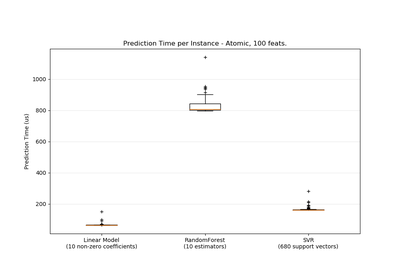Prediction Latency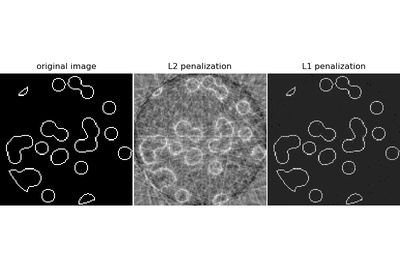Compressive sensing: tomography reconstruction with L1 prior (Lasso)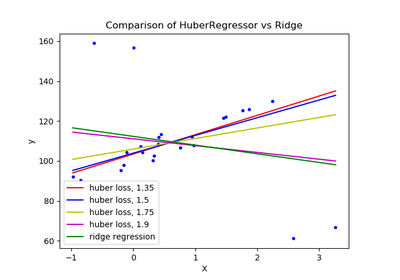HuberRegressor vs Ridge on dataset with strong outliers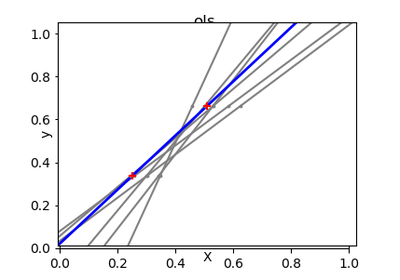Ordinary Least Squares and Ridge Regression Variance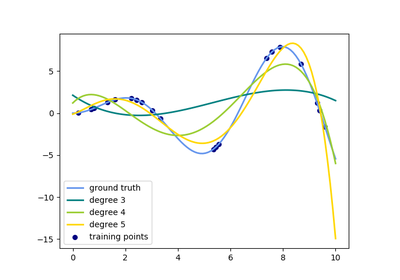Polynomial interpolation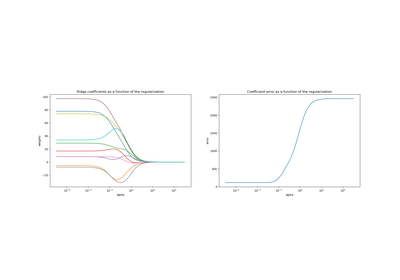Plot Ridge coefficients as a function of the L2 regularization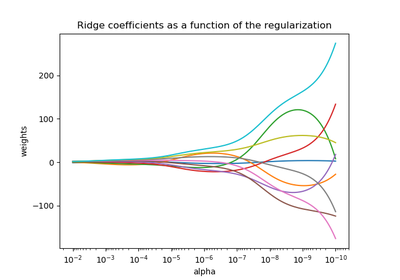Plot Ridge coefficients as a function of the regularization